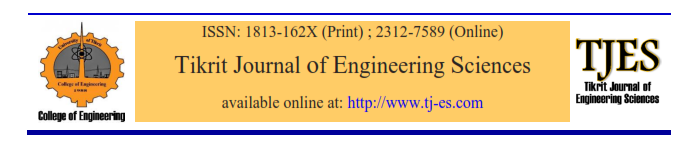# vol27no3pa4

TJES: Hussein TA, Sheet II. Implementation of Selective Harmonics Elimination for Single Phase Inverter using Arduino and Simulink MATLAB model. Tikrit Journal of Engineering Sciences 2020; 27(3): 31- 37.

APA: Hussein, T. A., Sheet, I. I. (2020). Implementation of Selective Harmonics Elimination for Single Phase Inverter using Arduino and Simulink MATLAB model. Tikrit Journal of Engineering Sciences, 27 (3), 31- 37.

References

 Muhammad H. .Power electronics: circuits, devices and systems (4th edition), Pearson / Prentice Hall, 2004).

 O. Bouhali, F. Solving Harmonic Elimination equations in multi-level inverters by using neural networks, International Journal of Information and electronics engineering. March 2013 vol.3, No.2.

 Jian Sun,. Solving nonlinear equations for selective harmonic eliminated PWM using predicted initial values, proceeding of the 1992 international conference on Industrial electronics, date added to IEEE explore, 06 August 2002

 Erwin k. Advanced engineering mathematics 10th edition, John Wiley & sons Inc. 2011.

 Lucian A. Selective harmonic elimination PWM Mathworks.com /matlabcentral /file exchange sept, 2017.

 Tamal B. Arduino UNO based packed U cell inverter for photovoltaic application, International symposium conference on devices, circuits and systems (ISDCS), March 2018.

 Maswood, A.I. et al. ‘A flexible way to generate PWM-SHE switching pattern using genetic algorithm’, IEEE Applied Power Electronics (APEC) Conf. Proc., Anaheim, California, USA, 2001 Vol. 2, pp.1130–1134.

 Sayyah, A. et al. ‘Optimization of THD and suppressing certain order harmonics in PWM inverters using genetic algorithms’, Proc. of IEEE International Symposium on Intelligent Control, Germany, Oct 2006 ., pp.874–879.

 Sundareswaran, K. et al. ‘Inverter harmonic elimination through a colony of continuously exploring ants’, IEEE Trans. Ind. Elect., 2007 Vol. 54, No. 5, Oct 2016, pp.2558–2565

 Wells, J.R. et al. ‘Modulation-based harmonic elimination’, IEEE Trans. Power Electron. 2007 Vol. 22, No. 1, Jan., pp.336–340.

 G. Rajpriga. Design and development of MATLAB Simulink based Selective Harmonic Elimination technique for three phase voltage source inverter, IEEE international conference on advanced computing and communication systems (ICACCS) , Dec 2013.

Tikrit Journal of Engineering Sciences (2020) 27(3): 31- 37.Implementation of Selective Harmonics Elimination for Single Phase Inverter using Arduino and Simulink MATLAB model

0 Mosul Technical College /Northern Technical University /Iraq

* Corresponding author: taha_hussian@yahoo.com

Abstract

This paper presents Arduino controller implementation and Simulink model in MATLAB to eliminate a selected number of harmonics in a single phase inverter. A MATLAB code is written to solve the nonlinear trigonometric that arises in the Fourier analysis of the output of the inverter. Newton’s Raphson method is adopted to find the triggering angles for the four electronic switches in the inverter. All possible combinations of these triggering instants are then computed for period of one cycle. To validate the model a practical set using Arduino controller is implemented. Frequency spectrums of the output of the inverter for both the Simulink model and the practical set are shown for the elimination of certain selected harmonics. Any other selected harmonics can be eliminated using the proposed model.

 Full Text PDF 148 Downloads

Keywords: Inverters, harmonic elimination, PWM, Newton’s Raphson, Simulink.

## Related ArticlesLoading...Taking too long?Reload document
|Open in new tab

Download [874.45 KB]